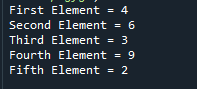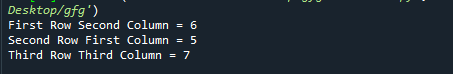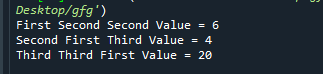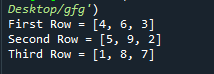# How to Reference Elements in an Array in Python

• Difficulty Level : Basic
• Last Updated : 03 Jan, 2021

Prerequisites: Numpy

Elements of an array can be referenced like a regular python array. Since python internally doesn’t support arrays, here whenever we use the term array we are referring to pythons list that can be used to build an array of any required dimension. Besides this, Python’s NumPy module also provides a container named ‘array’ for storing collections of data.  In this article, we will talk about how to reference elements in a Python array as well as numpy array in Python.

Attention geek! Strengthen your foundations with the Python Programming Foundation Course and learn the basics.

To begin with, your interview preparations Enhance your Data Structures concepts with the Python DS Course. And to begin with your Machine Learning Journey, join the Machine Learning - Basic Level Course

• For array referencing only the index of the required element has to be passed to the name of the array.

Syntax:

`array_name[index]`
• For referencing using numpy array, first an array is created using numpy’s array function then it is referenced like a regular array.

Syntax:

`np.array([array elements])`

Implementation using both methods is given below for various cases:

Example 1: Referencing items in a 1-D array

Example with Python Array

## Python3

 `# Creating a array of elements``arr ``=` `[``4``, ``6``, ``3``, ``9``, ``2``]`` ` `# Referring elements of the array``# by index to a new variable``first_element ``=` `arr[``0``]``second_element ``=` `arr[``1``]``third_element ``=` `arr[``2``]``fourth_element ``=` `arr[``3``]``fifth_element ``=` `arr[``4``]`` ` `# Print the variables``print``(``"First Element ="``, first_element)``print``(``"Second Element ="``, second_element)``print``(``"Third Element ="``, third_element)``print``(``"Fourth Element ="``, fourth_element)``print``(``"Fifth Element ="``, fifth_element)`

Output:Example with Python’s numpy module’s array

## Python3

 `# Importing numpy module``import` `numpy as np`` ` `# Creating a numpy array of elements``arr ``=` `np.array([``4``, ``6``, ``3``, ``9``, ``2``])`` ` `# Referring elements of the array``# by index to a new variable``first_element ``=` `arr[``0``]``second_element ``=` `arr[``1``]``third_element ``=` `arr[``2``]``fourth_element ``=` `arr[``3``]``fifth_element ``=` `arr[``4``]`` ` `# Print the variables``print``(``"First Element ="``, first_element)``print``(``"Second Element ="``, second_element)``print``(``"Third Element ="``, third_element)``print``(``"Fourth Element ="``, fourth_element)``print``(``"Fifth Element ="``, fifth_element)`

Output:Example 2: Referencing Items in a 2-D Array

Example with Python Array

## Python3

 `# Creating a 2d-array of elements``arr ``=` `[[``4``, ``6``, ``3``],``       ``[``5``, ``9``, ``2``],``       ``[``1``, ``8``, ``7``]]`` ` `# Referring elements of the 2d-array``# by row and column index to new variables``first_row_second_column ``=` `arr[``0``][``1``]``second_row_first_column ``=` `arr[``1``][``0``]``third_row_third_column ``=` `arr[``2``][``2``]`` ` `# Print the variables``print``(``"First Row Second Column ="``, first_row_second_column)``print``(``"Second Row First Column ="``, second_row_first_column)``print``(``"Third Row Third Column ="``, third_row_third_column)`

Output:Example with Python’s numpy module’s array

## Python3

 `# Importing numpy module``import` `numpy as np`` ` `# Creating a 2d-numpy-array of elements``arr ``=` `np.array([[``4``, ``6``, ``3``],``                ``[``5``, ``9``, ``2``],``                ``[``1``, ``8``, ``7``]])`` ` `# Referring elements of the 2d-array``# by row and column index to new variables``first_row_second_column ``=` `arr[``0``][``1``]``second_row_first_column ``=` `arr[``1``][``0``]``third_row_third_column ``=` `arr[``2``][``2``]`` ` `# Print the variables``print``(``"First Row Second Column ="``, first_row_second_column)``print``(``"Second Row First Column ="``, second_row_first_column)``print``(``"Third Row Third Column ="``, third_row_third_column)`

Output:Example 3: Referencing Items in a3-D Array

Example with Python array

## Python3

 `# Creating a 3d-array of elements``arr ``=` `[[[``4``, ``6``, ``3``], [``2``, ``6``, ``8``], [``3``, ``5``, ``12``]],``       ``[[``32``, ``11``, ``4``], [``23``, ``53``, ``89``], [``19``, ``17``, ``10``]],``       ``[[``14``, ``22``, ``52``], [``56``, ``43``, ``99``], [``20``, ``37``, ``32``]]]`` ` `# Referring elements of the 3d-array``# by 3d index to new variables``first_second_second ``=` `arr[``0``][``1``][``1``]``second_first_third ``=` `arr[``1``][``0``][``2``]``third_third_first ``=` `arr[``2``][``2``][``0``]`` ` `# Print the variables``print``(``"First Second Second Value ="``, first_second_second)``print``(``"Second First Third Value ="``, second_first_third)``print``(``"Third Third First Value ="``, third_third_first)`

Output:Example with Python’s numpy module’s array

## Python3

 `# Importing numpy module``import` `numpy as np`` ` `# Creating a 3d-array of elements``arr ``=` `np.array([[[``4``, ``6``, ``3``], [``2``, ``6``, ``8``], [``3``, ``5``, ``12``]],``                ``[[``32``, ``11``, ``4``], [``23``, ``53``, ``89``], [``19``, ``17``, ``10``]],``                ``[[``14``, ``22``, ``52``], [``56``, ``43``, ``99``], [``20``, ``37``, ``32``]]])`` ` `# Referring elements of the 3d-array``# by 3d index to new variables``first_second_second ``=` `arr[``0``][``1``][``1``]``second_first_third ``=` `arr[``1``][``0``][``2``]``third_third_first ``=` `arr[``2``][``2``][``0``]`` ` `# Print the variables``print``(``"First Second Second Value ="``, first_second_second)``print``(``"Second First Third Value ="``, second_first_third)``print``(``"Third Third First Value ="``, third_third_first)`

Output:Example 4: Referencing an Entire Row of an Array

Example with Python array

## Python3

 `# Creating a 2d-array of elements``arr ``=` `[[``4``, ``6``, ``3``],``       ``[``5``, ``9``, ``2``],``       ``[``1``, ``8``, ``7``]]`` ` `# Referring rows of the 2d-array``# by row index to new variables``first_row ``=` `arr[``0``]``second_row ``=` `arr[``1``]``third_row ``=` `arr[``2``]`` ` `# Print the variables``print``(``"First Row ="``, first_row)``print``(``"Second Row ="``, second_row)``print``(``"Third Row ="``, third_row)`

Output:Example with Python’s numpy module’s array

## Python3

 `# Importing numpy module``import` `numpy as np`` ` `# Creating a 2d-numpy-array of elements``arr ``=` `np.array([[``4``, ``6``, ``3``],``                ``[``5``, ``9``, ``2``],``                ``[``1``, ``8``, ``7``]])`` ` `# Referring rows of the 2d-array``# by row index to new variables``first_row ``=` `arr[``0``]``second_row ``=` `arr[``1``]``third_row ``=` `arr[``2``]`` ` `# Print the variables``print``(``"First Row ="``, first_row)``print``(``"Second Row ="``, second_row)``print``(``"Third Row ="``, third_row)`

Output:My Personal Notes arrow_drop_up